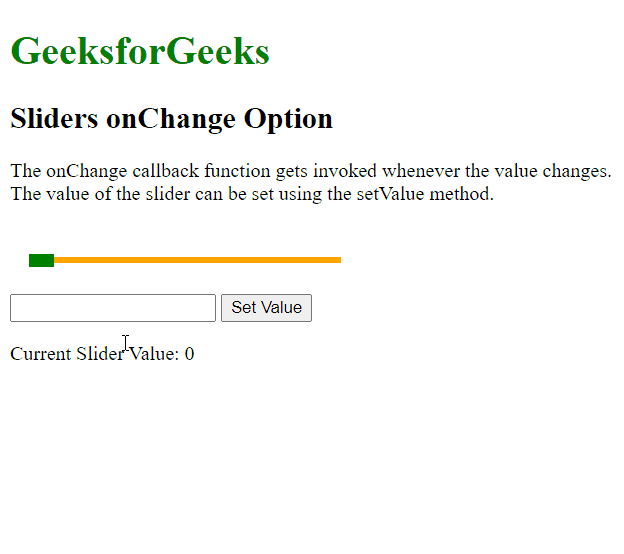# script.aculo.us Sliders onChange Option

• Last Updated : 30 Dec, 2020

The script.aculo.us library is a cross-browser library that aims at improving the user interface of a website. The Slider controls are thin tracks that allow the user to input values. It is done by defining a range of values that can be selected by the user by dragging the handle to the appropriate value.

The onChange option is used to specify a callback function that would be invoked whenever the value of the slider changes, either when the slider has finished moving or using the setValue() method to set a new value. The current value of the slider would be passed as a parameter to the function.

Syntax:

`{ onChange: function }`

Parameters: This option has a single value as mentioned above and described below:

• function: This is a callback function that would be invoked whenever the slider has finished moving or has been changed using the setValue() method.

The below example illustrates the use of this option.

Example:

## HTML

 ```<``html``>``<``head``>``  ````  ``<``script` `type``=``"text/javascript"` `          ``src``=``"prototype.js"``>``  ````  ``<``script` `type``=``"text/javascript"``          ``src``=``"scriptaculous.js?load = slider"``>``  ```` ` `  ````  ``<``style` `type``=``"text/css"``>``    ``.track {``      ``width: 250px;``      ``background-color: orange;``      ``height: 5px;``      ``position: relative;``    ``}`` ` `    ``.track .handle {``      ``width: 20px;``      ``height: 10px;``      ``background-color: green;``      ``cursor: move;``      ``position: absolute;``      ``top: -2px;``    ``}`` ` `    ``.pad {``      ``padding: 25px 15px;``    ``}``  ``````<``body``>``   ` `<``p``>``  ``<``h1` `style``=``"color: green;"``>``    ``GeeksforGeeks``  ````  ``<``h2``>Sliders onChange Option``   ` `<``p``>``    ``The onChange callback function ``    ``gets invoked whenever the value``    ``changes. The value of the slider ``    ``can be set using the setValue method.``  ```` ` ` ` `  ``<``div` `class``=``"pad"``>``    ``<``div` `id``=``"track-hor"` `class``=``"track"``>``      ``<``div` `id``=``"handle-hor"` `class``=``"handle"``>``      ````    ````  ```` ` `  ``<``input` `type``=``"text"` `id``=``"val"``>``  ``<``button` `onclick``=``"setVal()"``>``    ``Set Value``  ```` ` `   ` `<``p``>Current Slider Value: ``    ``<``span` `id``=``"out"``>0``  ```` ` ` ` `  ``<``script` `type``=``"text/javascript"``>`` ` `    ``// Initialize the slider``    ``let slider = new Control.Slider('handle-hor',``      ``'track-hor', {``       ` `      ``// Define the range``      ``range: \$R(1, 100),`` ` `      ``onChange: function (currValue) {``        ``document.querySelector("#out")``                  ``.textContent = currValue;``      ``}``    ``});`` ` `    ``function setVal() {`` ` `      ``// Get the value form the input box``      ``let val =``          ``document.querySelector("#val")``                    ``.value;`` ` `      ``// Set the value of the slider``      ``// to the given value``      ``slider.setValue(val);``    ``}``  ```````

Output:My Personal Notes arrow_drop_up